Inverse Trigonometric Functions MathBitsNotebook.com Terms of Use   Contact Person: Donna RobertsWhen working with trigonometric inverse problems, keep the following information in mind:

 Inverse Notation: arcsin(x) = sin-1(x) arccos(x) = cos-1(x) arctan(x) = tan-1(x) arcsin(x)is read "the angle whose sine is x".Reference Triangles: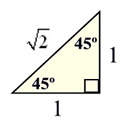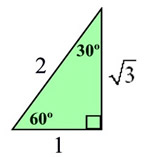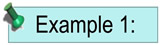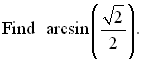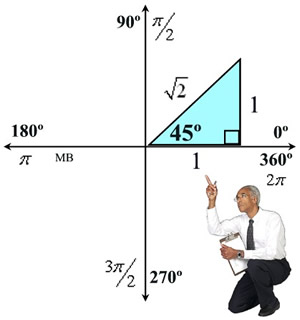Solution: You are looking for "the angle whose sine is"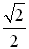and the angle is in the interval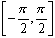. Sine is positive in Quadrant I. Yes, sine is also positive in Quadrant II, but Quadrant II is not used to create sine inverse.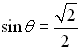and we know that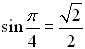, so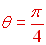or 45º.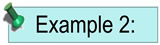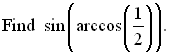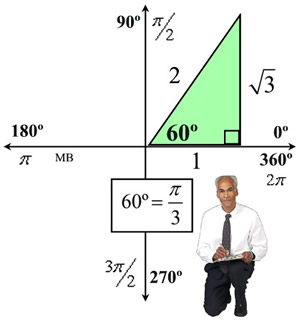Solution: You are looking for the sine of "an angle whose cosine is" 1/2 in the interval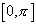.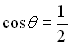and we know that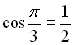, so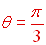.  Cosine is positive in Quadrant I. Now, finish the question by finding the sine of this result: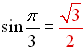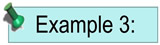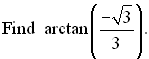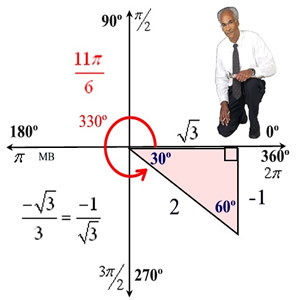Solution: You are looking for "the angle whose tangent is"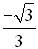and the angle is in the interval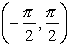.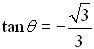and we know that a reference angle of 30 degrees in quadrant IV will set up our situation.  We need to remember that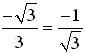.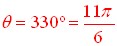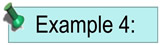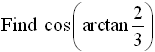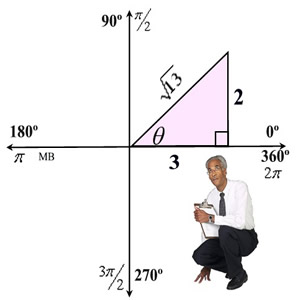Tangent is positive in Quadrant I. Solution: You are looking for "the angle whose tangent is"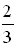and the angle is in the interval.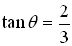creates the triangle shown at the right. The Pythagorean Theorem was used to find the length of the hypotenuse.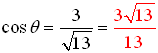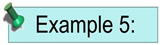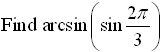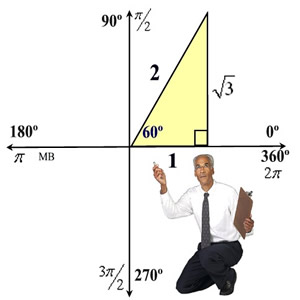Sine is positive in Quadrant I. Solution: You are looking for "the angle whose sine is" the same as the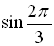in the interval.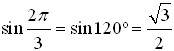You need to find an angle whose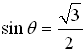in.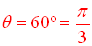Remember when f (f-1(x)) = x? This concept does not apply in this situation because of the restrictions on the domain of sine inverse.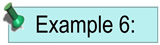Use the inverse function of sine, cosine, or tangent to solve this problem: Given: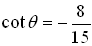and cos θ > 0, find the exact value of sin θ.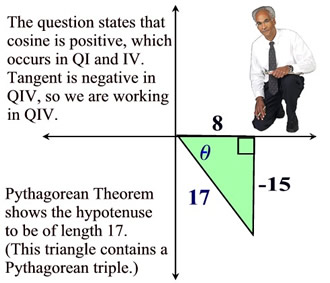Since this question asks for "exact value", the calculator cannot be used to give a decimal approximation. Answer: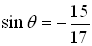Solution: To solve this problem we need to know the "angle whose" cotangent is -8/15, which would imply the use of the inverse of cotangent. But, our question specifies the use of the inverse of sine, cosine or tangent only.  Let's rewrite the first given condition to be: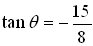and use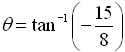Our problem is to now find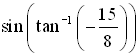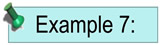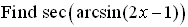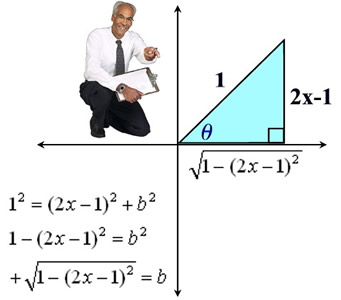Solution: You are looking for secant of "the angle whose sin is" 2x-1 in the interval. From labeling the triangle and using the Pythagorean Theorem, we can read the secant of the angle.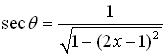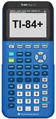For help with trigonometric inverses on your calculator, click here.